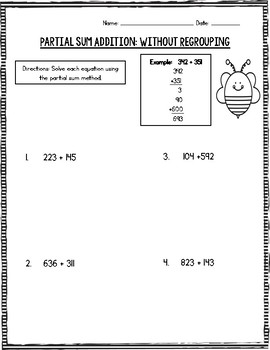# Partial Sum Triple Digit Addition Pack1st - 3rd
Subjects
Standards
Resource Type
Formats Included
• PDF
Pages
19 pages

### Description

Addition with multiple methods and deeply understanding numbers is a big part of the Common Core. This product is perfect to help students learn another method to add two triple digit numbers, by breaking them down into hundreds, tens, and ones using the partial sum method.

If you haven't introduced the partial sum method yet, make sure you start with double digit addition using partial sum first!

This product includes:

-3 mini anchor charts/posters (partial sum, partial sum addition without regrouping, partial sum addition with regrouping)

-2 worksheets for triple digit addition (using the partial sum method) without regrouping and answer keys

-2 worksheets for triple digit addition (using the partial sum method) with regrouping and answer keys

-2 assessments (one without regrouping and one with regrouping) and answer keys

-one cut/paste sorting activity for partial sum of triple digit numbers

Common Core Standards:
2OAB2
2NBTB7
2NBTB6

Be sure to check out my other math products:

Common Core Decomposing Numbers Sorting Fun

Partial Sum Holiday Pack

Total Pages
19 pages
N/A
Teaching Duration
N/A
Report this Resource to TpT
Reported resources will be reviewed by our team. Report this resource to let us know if this resource violates TpT’s content guidelines.

### Standards

to see state-specific standards (only available in the US).
Fluently add and subtract within 20 using mental strategies. By end of Grade 2, know from memory all sums of two one-digit numbers.
Add up to four two-digit numbers using strategies based on place value and properties of operations.
Add and subtract within 1000, using concrete models or drawings and strategies based on place value, properties of operations, and/or the relationship between addition and subtraction; relate the strategy to a written method. Understand that in adding or subtracting three-digit numbers, one adds or subtracts hundreds and hundreds, tens and tens, ones and ones; and sometimes it is necessary to compose or decompose tens or hundreds.